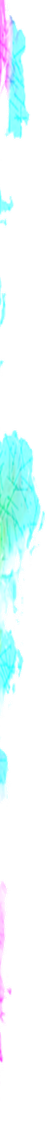### Text

To draw a text

• turned_in_notText Related Tags
• `<text>`
• `<tspan>`
• `<textPath>`

##### <text>

Used to draw a text on svg, default fill color is black

Full Syntax

``````<text x="p1 p2 p3 .... pN"
y="p1 p2 p3 .... pN"
dx="d1 d2 d3 ... dN"
dy="d1 d2 d3 ... dN"
rotate="r1 r2 ... rN"
textLength="length"
</text>``````
• label_outlinetext Attributes
• x - List of start points of Nth-index character at x-axis. the reaming text (if any) follow the last defined Nth-index character
• y - List of start points of Nth-index character at y-axis. the reaming text (if any) follow the last defined Nth-index character
• dx - List of distances which moves Nth-index character relative to the (N-1)th-index character along x-axis
• dy - List of distances which moves Nth-index character relative to the (N-1)th-index character along y-axis
• rotate - List of rotation angles of every Nth-index character, the last rotation angle will set to all reaming text (if any) after the defined Nth-index
• textLength - The total length text will take
• lengthAdjust - Possible values are "spacing | spacingAndGlyphs"

Syntax - text character start at specific point

``&lt;text x="p1 p2 p3 ... pN" y="p1 p2 p3 ... pN"> Your Text &lt;/text>``
The 1st character start at (50,50), 2nd character start at (50,60) others reaming follow the 2nd character
``````<svg height="100" width="200">
<text x="50" y="50 60" style="fill:red">Hello World</text>
</svg>``````
text

Syntax - text with specific distance between characters

``&lt;text dx="d1 d2 d3 ... dN" dy="d1 d2 d3 ... dN"> Your Text &lt;/text>``

*The black text showing the actual position of text in below examples

The 1st character moves 10 unit from its last position & 2nd character moves 20 unit from now 1st character position, along x-axis
``````<svg height="100" width="200">
<text x="50" y="50" style="font-size:30px">Hello</text>
<text x="50" y="50" dx="10 20" style="fill:red;font-size:30px">Hello</text>
</svg>``````
text(With dx)

The 1st character moves 10 unit from its last position & 2nd character moves 30 unit from now 1st character position, along y-axis
``````<svg height="100" width="200">
<text x="50" y="50" style="font-size:30px">Hello</text>
<text x="50" y="50" dy="10 30" style="fill:red;font-size:30px">Hello</text>
</svg>``````
text(With dy)

Syntax - text with specific rotated characters

``<text rotate="r1 r2 ... rN"> Your Text </text>``
The 1st character rotated at 10deg, 2nd character rotated at 40deg and others after, rotated at 80deg
``````<svg height="100" width="200">
<text x="50" y="50" rotate="10 40 80" style="fill:red;font-size:30px">ABCDE</text>
</svg>``````
text(With rotate)

Syntax - text within specific length

``<text textLength="length"> Your Text </text>``
The text length is set to 180px
``````<svg height="100" width="200">
<text x="10" y="50" textLength="180" style="fill:red;font-size:30px">Hello</text>
</svg>``````
text(With textLength)

Syntax - text layout at specific length

``<text textLength="length" lengthAdjust="spacing | spacingAndGlyphs"> Your Text </text>``
The text length is set to 180px
``````<svg height="100" width="200">
<text x="10" y="40" textLength="180" lengthAdjust="spacing" style="fill:red;font-size:20px">Hello</text>
<text x="10" y="80" textLength="180" lengthAdjust="spacingAndGlyphs" style="fill:red;font-size:20px">Hello</text>
</svg>``````

##### <tspan>

Used inside text tag or inside itself (nested), default fill color is black

Full Syntax - similar as of text tag

``````<tspan x="p1 p2 p3 .... pN"
y="p1 p2 p3 .... pN"
dx="d1 d2 d3 ... dN"
dy="d1 d2 d3 ... dN"
rotate="r1 r2 ... rN">
</tspan>``````
• label_outlinetspan Attributes
• x - List of start points of Nth-index character at x-axis. the reaming text (if any) follow the last defined Nth-index character
• y - List of start points of Nth-index character at y-axis. the reaming text (if any) follow the last defined Nth-index character
• dx - List of distances which moves Nth-index character relative to the (N-1)th-index character along x-axis
• dy - List of distances which moves Nth-index character relative to the (N-1)th-index character along y-axis
• rotate - List of rotation angles of every Nth-index character, the last rotation angle will set to all reaming text (if any) after the defined Nth-index
The tspan tag is nested inside text tag and start from point(60,70)
``````<svg height="100" width="200">
<text x="50" y="50 60" style="fill:red">Hello
<tspan x="60" y="70" style="fill:blue">World</tspan>
</text>
</svg>``````
tspan

##### <textPath>

Used inside text tag, to show any text along any svg component path

Full Syntax

``````<textPath xlink:href="anyComponentPathId" startOffset="number">
</textPath>``````
• label_outlinetextPath Attributes
• xlink:href - Reference of component path to which text follows
• startOffset - Distance after which text to show on component path, from starting point of the path
The text follows the circular path
``````<svg height="100" width="200">
<circle cx="100" cy="50" r="40" stroke="black" fill="none"  id="circlePath"/>
<text style="fill:red">